Reach Us+44-1625-708989
Control of Laminar Fluid Flow and Heat Transfer in a Planar T-Channel with Rotating Obstacle | OMICS International
Journal of Physical Mathematics
All submissions of the EM system will be redirected to Online Manuscript Submission System. Authors are requested to submit articles directly to Online Manuscript Submission System of respective journal.

# Control of Laminar Fluid Flow and Heat Transfer in a Planar T-Channel with Rotating Obstacle

Palraj Jothiappan*

Department of Mathematics, PSG College of Arts and Science, Coimbatore, Tamil Nadu, India

*Corresponding Author:
Palraj Jothiappan
Department of Mathematics
PSG College of Arts and Science Coimbatore, India
Tel: 0422 430 3300
E-mail: [email protected]

Received Date: May 15, 2015; Accepted Date: June 09, 2015; Published Date: July 15, 2015

Citation: Jothiappan P (2015) Control of Laminar Fluid Flow and Heat Transfer in a Planar T-Channel with Rotating Obstacle. J Phys Math 6:142. doi:10.4172/2090-0902.1000142

Copyright: © 2015 Jothiappan P. This is an open-access article distributed under the terms of the Creative Commons Attribution License, which permits unrestricted use, distribution, and reproduction in any medium, provided the original author and source are credited.

Visit for more related articles at Journal of Physical Mathematics

#### Abstract

In the present study, a laminar flow in a planar 2D right angled T-channel in the presence of a rotating heated cylindrical obstacle placed in the junction area is numerically studied to control the heat transfer and fluid flow. The effect of Reynolds number (20 ≤ Re ≤ 300) and cylinder rotation angle (-5 ≤ Ã‰Â· ≤ 5) on the fluid flow and heat transfer characteristics are studied numerically. It is observed that the flow field and heat transfer rate are influenced by the variation of these parameters.

#### Keywords

Finite element method; Navier-Stokes equation; Rotating obstacle; Rotating cylinder; T-channel; Recirculation length; Heat transfer

#### Nomenclature

c: Temperature, K

Cp: Specific heat, J/kg K

D: Diameter of the cylinder, m

H: Width of the branch, m

h: Local heat transfer coefficient, Wm-2K-1

k: Thermal conductivity, Wm-1K-1

L1: Upstream length of main branch, m

L2: Downstream length of main branch, m

L3: Side branch length, m

n: Unit normal vector

Nu: Local Nusselt number, hH/k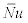: Spatial-averaged Nusselt number

P: Pressure, Pa

Pr Prandtl number, μCp/k

Re: Reynolds number, UH/v

t: Time, s

u: X-component velocity, ms-1

v: Y-component velocity, ms-1

x,y: Cartesian coordinates, m

Greek Symbols

ρ : Density, kgm-3

ν : Kinematic viscosity, m2 s-1

ɷ: Cylinder rotation angle.

#### Introduction

The incompressible Navier-Stokes system is one of the main equations studied in mathematical physics and fluid mechanics fields . The Navier Stokes equations describe the flow of incompressible, newtonian fluids. The equations are transient, nonlinear and velocity is non-trivially coupled with pressure. A lot of research has been devoted to finding efficient ways of linearzing, coupling and solving these equations. Therefore, to utilize the computational power of modern high-performance computers, much effort is thrown into the development of efficient computing methods for the Navier-Stokes equations [2-5]. FEniCS is a generic open source software framework that aims at automating the discretization of differential equations through the finite element method [6,7]. The three most common flow configurations are branching T-channel, impacting T-channel and combining T-channel. The present study is concerned with the flow in branching T-channel. Branching fluid flow and heat transfer in a 90 degree T-channel is of considerable importance to a wide variety of applications in the biomedical and engineering fields. Flow through a T-channel has a wide range of applications, such as biomechanical applications, phase separation, oil and gas pipelines, polymer and pharmaceutical industries, irrigation systems, wastewater treatment, ventilation systems and in many other areas. Boundary-layer development, flow separation, and secondary flows that occur in these complex flows give rise to significant modification of heat and mass transport, as reported by various experimental groups see [8,9]. Heat transfer and fluid flow characteristics over a backward facing [10,11] and forward facing  step in a channel with the insertion of rotating obstacles has received some attention in the literature [13,14].

The mechanics of such flow are complex and not well understood exhibiting nontrivial flow patterns which include zones of recirculation and stream wise vortices. The distribution of the flow into various branches depends on the flow resistances of these branches and in general, it is even impossible to predict the direction of flow through branches under given pressure drops. It has been a geometrical model of choice because in addition to its simplicity, its flow features demonstrate the most common flow behaviour at arterial bifurcations. Pollard has experimentally investigated the laminar and turbulent fluid flow and heat transfer in T channel prior to 1978 . Kawashima et al. experimentally studied the turbulent heat transfer in a two-dimensional right-angled confluence . They observed that the axial variation of the local Nusselt number was affected by the cross-sectional area and flow rate ratios, but was affected insignificantly by the Reynolds number. Hayes et al.  studied the steady laminar flow in a 90 degree planar branch; they found that the fractional flow in the main duct increases with increasing Reynolds number for the case of constant exit pressures at the outlet of each branch. Neary and Sotiropoulos presented numerical solutions for the steady 3-D laminar flows through a 90 rectangular cross section . They compared solutions with experimental measurements to elucidate the flow topology patterns and showed that both length and width of the separation zone decrease with increasing discharge ratio. Dhiman investigated the flow characteristics of non-Newtonian power-law fluids in a right-angled horizontal T-channel in the laminar regime . They observed that for a particular power-law index, the length of recirculation zone increases in the side branch with increasing Reynolds number. Hassan and Kim studied numerically the mixing of a high-pressure coolant injected into the leg of a pressurized water reactor during the loss of coolant accident . Sparrow investigated the Effect of a Mixing Tee on turbulent heat transfer in a tube . The mixing of the two streams of air gave rise to a remarkable augmentation of the heat transfer coefficient compared with those in a conventional thermal entrance region.

Thus, based on the above discussion, flow in a T-channel for Newtonian and non-Newtonian fluids has been investigated extensively both experimentally and numerically to obtain the basic information of flow separation and reattachment phenomena in the laminar flow regime. The two-dimensional (2D) laminar flow for Newtonian fluids in a T-channel in the presence of a rotating heated circular cylinder placed in the junction is not investigated yet and the lack of such results motivated the current study. The 2-D simulations are deemed adequate to represent actual three-dimensional situations when the aspect ratios of the ducts forming the T-channel are large, as in the experiments of Liepsch  and Khodadadi . The main objective of this study is to investigate the characteristics of two-dimensional (2D) laminar flow for Newtonian fluids in a T-channel in the presence of a rotating heated circular cylinder placed in the junction area over a range of Reynolds numbers (20 ≤ Re ≤ 300) and cylinder rotation angle (-5 ≤ ɷ ≤ 5). To the best of author’s knowledge and based upon the above literature survey such a study has not been seen in the literature.

#### Numerical Simulations

Geometry of the computational domain

In this section, the computational domain and configurations of the obstacle are presented. A schematic description of the physical problem is shown in Figure 1a. All branches have the same width H. The inlet condition was set as fully developed with the corresponding velocity pro le having a parabolic shape. The minimum lengths of the bifurcation channels that guaranty a fully developed flow at the outlets depend on the flow characteristics. According to Shah and London  the pipe length required to develop a Newtonian flow is given by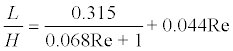It helps in estimating the required geometry dimensions. The Reynolds number is defined as: Re=UH/v where v is the viscosity of the fluid. The distance between the inlet plane and the junction of the channel L1 is taken as 5H, and the downstream distance between the junction and the exit plane L2 is taken as 20H. The length of side branch L3 is taken as 15H. The circular cylinder with diameter D=0.2 is kept at channel junction. The diameter of the cylinder is so chosen as to keep the H/D ratio in the appropriate range so as to ensure the steady nature of the flow in the downstream channel as has been previously described by Singha and Sinhamahapatra  for the channel flow. The distance from the centre of the cylinder to the inlet plane of the channel is 6H. The circular heated cylinder Γ9 is maintained at constant temperature higher than the inlet temperature. The wall boundaries Γ2457 and Γ8 of the channel are treated as no slip boundary with adiabatic condition. At the inlet of the channel Γ1 a parabolic velocity (U) and a uniform temperature are imposed and pressure is set to zero at the out flow boundaries Γ3 and Γ6 Working fluid is air with a Prandtl number Pr=0.71. The flow is assumed to be two dimensional, Newtonian, incompressible and in the laminar flow regime.

Governing equations and boundary conditions

The governing equations for two dimensional, incompressible, laminar and unsteady cases can be written as follows,

Continuity equation

Div u=0 (2.1)

Momentum equation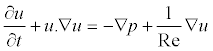(2.2)

Energy equation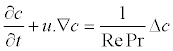(2.3)

Where c is temperature.

The boundary conditions are given as follows,

At the channel inlet Γ1, velocity is unidirectional parabolic and temperature is uniform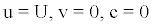(2.4)

On the channel walls Γ2457 and Γ8 adiabatic wall with noslip boundary conditions are assumed,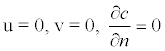(2.5)

Where n denote the surface normal directions

On the cylinder surface specified velocity components with constant temperature boundary condition is used

U=-ɷ(y-y0), v= ɷ(x-x0), and c=1

At the out flow boundary main branch Γ3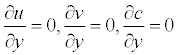(2.6)

At the out flow boundary side branches Γ6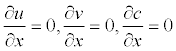(2.7)

Once the velocity and temperature fields are obtained, the local Nusselt number is defined as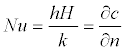(2.8)

Where h represents the local heat transfer coefficient and k denotes the thermal conductivity of air.

The spatial-averaged Nusselt number along the surface of a cylinder is calculated as

Follows: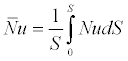(2.9)

Where S represents the surface area of the cylinder, n denote the surface normal directions of the cylinder.

The governing equations (2.1-2.3) along with the above boundary conditions (2.4-2.7) are solved for the fluid flow and heat transfer in a T-channel over a Rotating heated circular cylinder obstacle to obtain velocity, pressure and temperature Fields.

#### Finite Element Scheme and Implementation

To construct a time discretization scheme for the Navier-Stokes equations (2.1-2.3). Our choice is a Fully-implicit Crank-Nicolson scheme as described in  for retaining the basic non-linearity in the Navier-Stokes equations. The time centred Crank-Nicolson scheme gives nonlinear equations to be solved at each time level and redefining u be the velocity at the new time level and u1 be the velocity at the previous time level, we arrive at these spatial problems: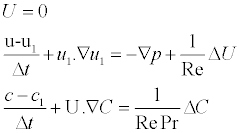With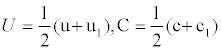Denoting the arithmetic averages needed in Crank-Nicolson time integration. The corresponding variation formulation involves the integrals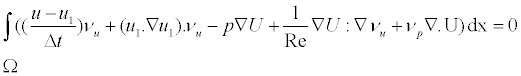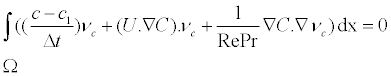(3.1)

Where vu; vp and vc are test functions for the test spaces of u; p; and c respectively.

Finite element software basically has to solve three sub problems: Mesh generation, system-matrix assembly and solution of the resulting linear systems of equations. We use Mshr for mesh generation and FEniCS  for matrix assembly and the solution of linear systems. CBC PDE Sys is built upon the finite element package FEniCS . FEniCS is a powerful development environment for performing finite element modelling, including strong support for symbolic automatic differentiation, native parallel support and parallel interface with linear algebra solvers such as PET Sc and Trillions, and automatic code generation and compilation for compiled performance from an interpreted language interface. The Python scripting environment makes the generation and linking of new code straightforward. A finite element method consisting of 12673 vertices and 23860 triangular elements as shown in Figure 1b, is used in this study. The mesh is finer near the walls of the channel and cylinder to resolve the higher gradients in the thermal and velocity boundary layer and in the vicinity of the junction. The sensitivity analysis of the simulation results with the number of elements, mesh size are also assured to obtain an optimal grid distribution with accurate results and minimal computational time. Three different grid sizes are tested and the convergence in the recirculation zone along the bottom wall downstream of the side branch is checked for Reynolds numbers 100 and w=0, tabulated in Table 1. Grid sensitivity analysis for Re=100 and ɷ=0.

Grid Size Lr=D Relative difference (%)
17979 2.8510 0.0947
23680 2.8532 0.0175
34719 2.8537 0.0000

Table 1: Grid sensitivity analysis for Re=100 and ɷ=0.

Validation study

The numerical solution procedure used here has been benchmarked with standard results for the incompressible flow of Newtonian fluids in a T-channel reported in the literature [15,17]. The Figure 2 shows the validation of reattachment length for Newtonian fluids on varying Reynolds numbers. The minimum deviation for the percentage in the error is obtained for the results of  is found to be about 0.31, whereas the maximum deviation is around 2.74. This validates the present numerical solution, the comparison results shows good overall agreement and slight differences for higher Reynolds number. This difference is believed to be due to the differences in the domain and grid sizes used by others as opposed to the present study.

#### Results and Discussion

The main parameters that affect the fluid flow and thermal characteristics are Reynolds number, Prandtl number, and distance between the inlet to the junction, cylinder position and diameter, expansion ratio and cylinder rotation angle. In the current study, the numerical simulations are performed for the Reynolds number (20 ≤ Re ≤ 300) and cylinder rotation angle (-5 ≤ ɷ ≤ 5) re-examined for fluid flow structures and convective heat transfer enhancement over a T-channel.

Effects of cylinder rotation angle

Figures 3 and 4 indicates the effect of varying cylinder rotation angle (ɷ) on the streamlines and isotherms at blockage ratio of 20 percentages for fixed values of Reynolds number 300 and Prandtl number 0.71. The case ɷ=0 corresponds to a stationary heated cylinder which is shown in Figures 3b, 3e and 3h. In this case, streamlines are slightly.

Defected towards the side branch in the presence of the motionless cylinder and several vortices appear behind the obstacle and in the vicinity of the cylinder close to the bottom wall of side branch. The case ɷ=0, no recirculation zone is occur in the side branch till the Re=18. The flow separation is delayed with cylinder rotation angle (ɷ). When the cylinder rotates in the clockwise direction (negative value of ɷ) (Figures 3a, 3d and 3g) flow is accelerated in the region between the left channel wall and the cylinder due to the contraction effect. Some portion of the flow is directed towards the right of the cylinder and related to this effect, the vortex appear on the upper right of the cylinder for motionless cylinder case disappears. The size and extent of the recirculation bubble appearing on the side branch bottom wall increase compared to motionless cylinder case. As it can be seen in the Figures 3a, 3d and 3g the extent and strength of the recirculation bubble can be controlled with cylinder rotation angle.

A positive value of the rotation (ɷ) indicates counter clockwise rotation of the cylinder (Figures 3c, 3f and 3i). When the cylinder rotates in this direction, more flow is accelerated towards the main branch due to the combined effect of contraction area and rotation. The motion of the fluid owing through the right of the cylinder and the size of the recirculation zone on the side branch upper wall are affected compared to motionless cylinder case. The flow structure near the upper and right of the cylinder are affected to some extent. The size and extent of the recirculation bubble appearing on the side branch bottom wall decreases compared to motionless cylinder case. Figure 4 shows the effect of cylinder rotation on the isotherms for Reynolds number Re=300. For counter clockwise rotation direction, the isotherms fluctuates more less on the side branch (Figures 4a and 4d) and right and bottom parts of the cylinder due to the formation of the vortices compared to motionless cylinder case. When the cylinder rotates in clockwise direction, the isotherms fluctuates more on the side branch and less clustering of the isotherms for the side branch due to the recirculation region and indicates poor heat transfer characteristic for this region since more flow is directed towards the spacing between the main branch left channel wall and cylinder. The case ɷ=5, the isotherms fluctuates both main and side branches, while comparing to the other cases. Local Nusselt number distributions along the surfaces of the circular cylinder are demonstrated in Figure 5b. Introducing a heated cylinder (motionless or rotating) enhances the thermal transport from the main branch and the side branch due to the flow acceleration towards the main and side branches. For the counter clockwise rotation of the cylinder, heat transfer is less effective on side branch. Since more flow is entrained into the side branch and defection of the flow patterns upwards on the top of the side branch. For clockwise rotation of the cylinder, better heat transfer characteristic is observed at surface of the side branch due to the flow acceleration towards the side branch.

Figure 5: (a) Variation of recirculation length Lr=H and (b) averaged Nusselt number with Reynolds number at Different values of cylinder rotation angle.

Wake length

The variation of the recirculation wake) length (Lr=H) in the side branch (defined as the distance from the junction of T- channel to the point of attachment of the fluid with the bottom wall) as a function of Reynolds number and angle of rotation ɷ is shown in Figure 5a. The length of the recirculation zone is seen to increase in a nonlinear fashion with an increase in Reynolds number for a particular angle of rotation. The recirculation length is also seen to increase with decreasing angle of rotation for a fixed Reynolds number. Thus, the dimensional considerations suggest that the recirculation length is a function of Reynolds number and angle of rotation.

Effects of Reynolds number

Figures 6 shows the effect of varying Reynolds numbers on the flow patterns at fixed values of !=5 and different time level. The fluid while travelling from main branch to side branch maintains contact with the wall of the side branch. As the Reynolds number is increased, beyond a critical point the fluid gets separated from the lower wall of the side branch and a closed recirculation region is developed.

Further downstream of the reattachment points, the flow regains its fully developed flow behaviour. With a gradual increase in the value of the Reynolds number (Re>18), the size of recirculation region increases. The flow patterns for the case of Newtonian fluids in a T-channel are found to be in close agreement with those of Hayes et al.  and Neary and Sotiropoulos . As Reynolds number increases, the flow begins to separates behind the cylinder causing vortex shedding which is an unsteady phenomenon and recirculation region is seen and increases in size and strength. At Reynolds number of 200, some portion of the fluid separated from the bottom wall and separates from the main branch side wall and again separates behind the cylindrical obstacle as seen in Figure 6. When Reynolds number increases, the flow separated from the bottom wall is entrained into the wake of the cylindrical obstacle and formation of the vortices is seen behind the cylindrical obstacle shown in Figures 6e and 6f. Figure 7 indicates the effect of varying Reynolds numbers on the isotherms for fixed value of rotation angle ɷ=5. When the cylinder rotates in this direction, the isotherms fluctuates more on the side branch and less clustering of the isotherms for the side branch due to the recirculation region and indicates better heat transfer characteristic for this region since more flow is directed towards the spacing between the main branch left channel wall and cylinder.

#### Conclusion

In this study, laminar flow in a planar right angled T-channel in the presence of a rotating heated circular cylinder placed in the junction area is numerically studied. The effect of Reynolds number (20 ≤ Re ≤ 300) and cylinder rotation angle (-5 ≤ ɷ ≤ 5) on the fluid flow and heat transfer characteristics are numerically investigated.

The numerical methodology has been extensively validated against previous numerical and experimental studies. The grid and computational domain were chosen after extensive testing by varying various grid densities. Detailed observations of flow pattern, recirculation length, local and averaged Nusselt number for the onset of flow separation and viscosity variation along the bottom wall of the side branch have been presented.

The result shows that the length of the recirculation zone increases on increasing Reynolds number for a particular angle of cylinder rotation. It also increases on decreasing the angle of cylinder rotation for a fixed value of Reynolds number. As the Reynolds number increases local Nusselt number also increases. When the cylinder rotates in the clockwise direction, more flow is entrained into the wake of the cylinder and some portion of the flow is directed towards the right of the cylinder and related to this effects the size and extent of the recirculation bubble appearing on the side branch lower wall and main branch side wall. Length and intensity of the recirculation zone behind the obstacle are considerably affected with the installation of the cylindrical obstacle and rotation angle. Adding the rotating cylindrical obstacle alters the isotherm plots. It is seen that there is significant change on the clustering of the isotherm patterns and the location where this steep temperature gradient occurs in the flow with the installation of the rotating cylindrical obstacle. The result shows the effect of rotation on the local Nusselt number distribution is more pronounced at low Reynolds number.

#### References

Select your language of interest to view the total content in your interested language

### Article Usage

• Total views: 14303
• [From(publication date):
November-2015 - Jul 20, 2019]
• Breakdown by view type
• HTML page views : 10251Can't read the image? click here to refresh